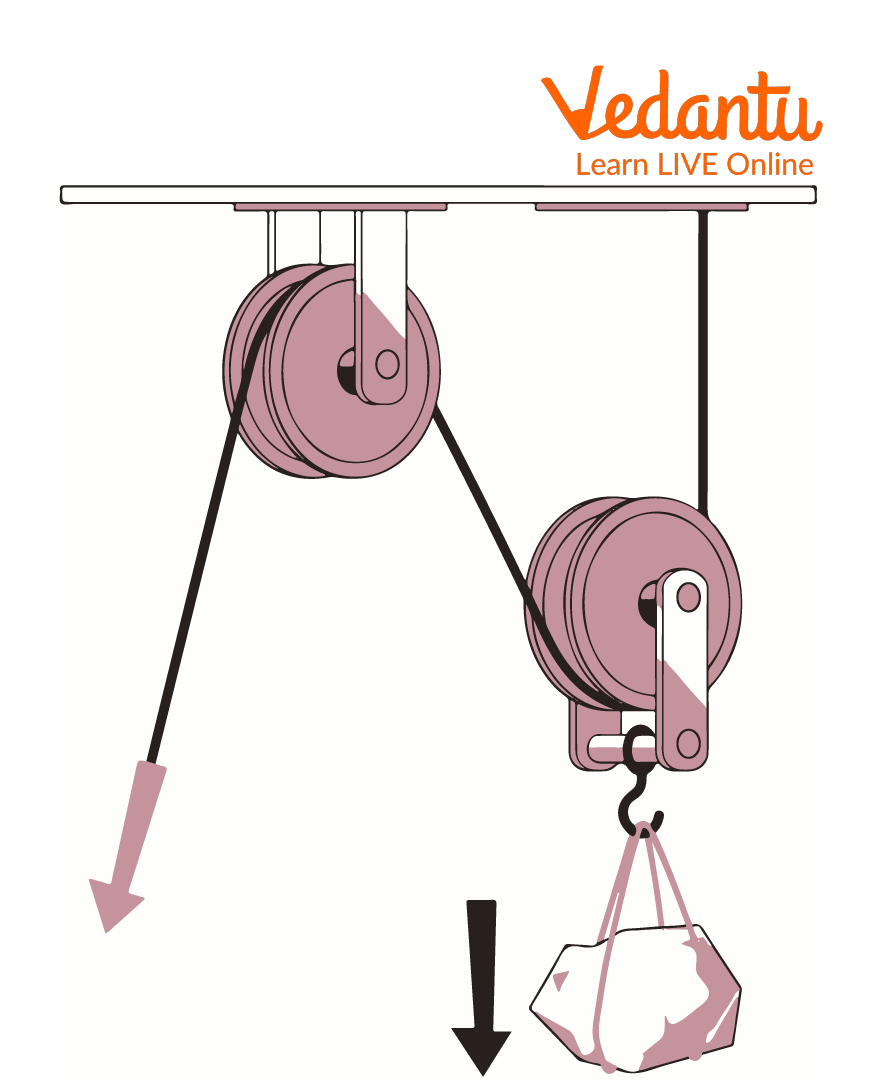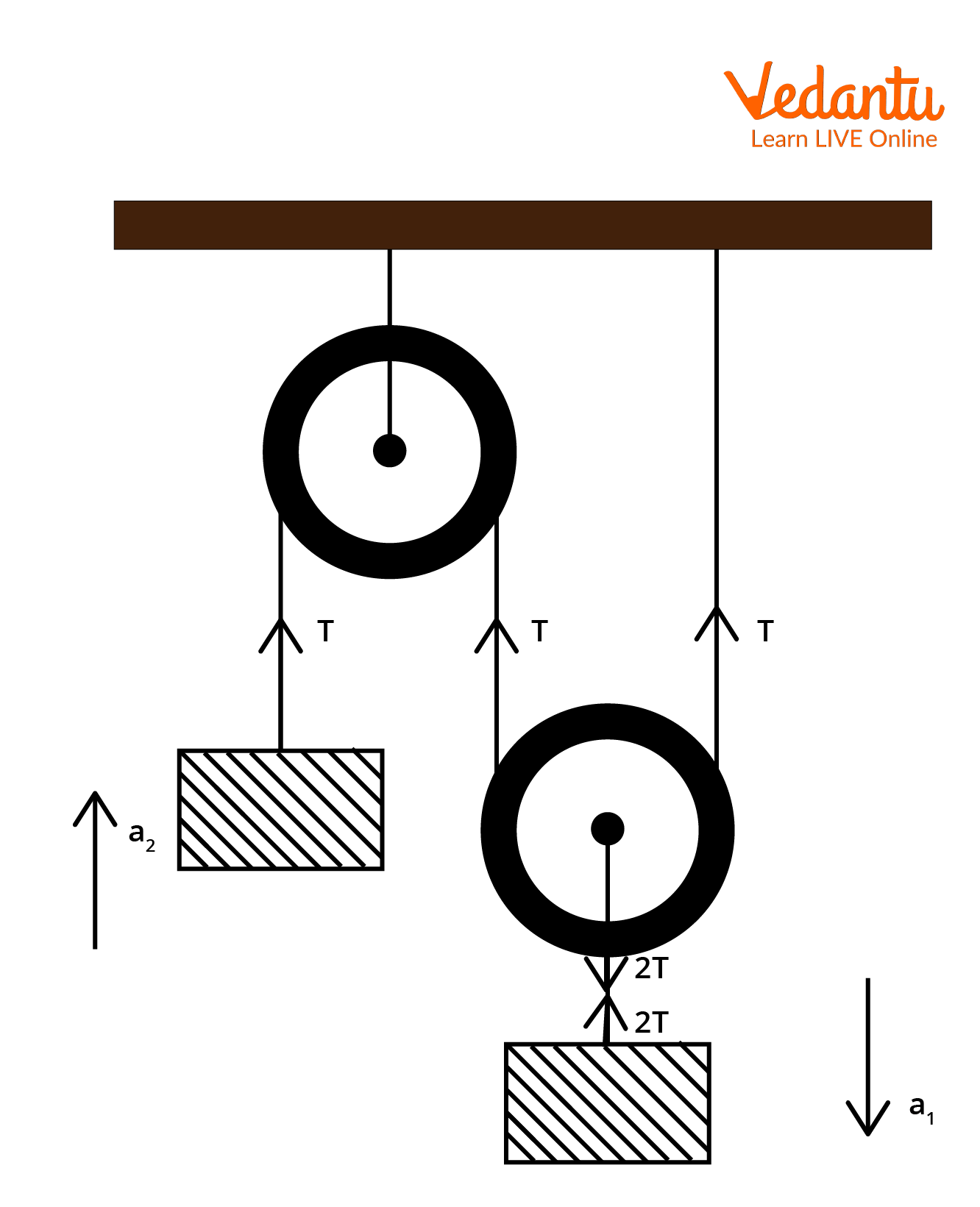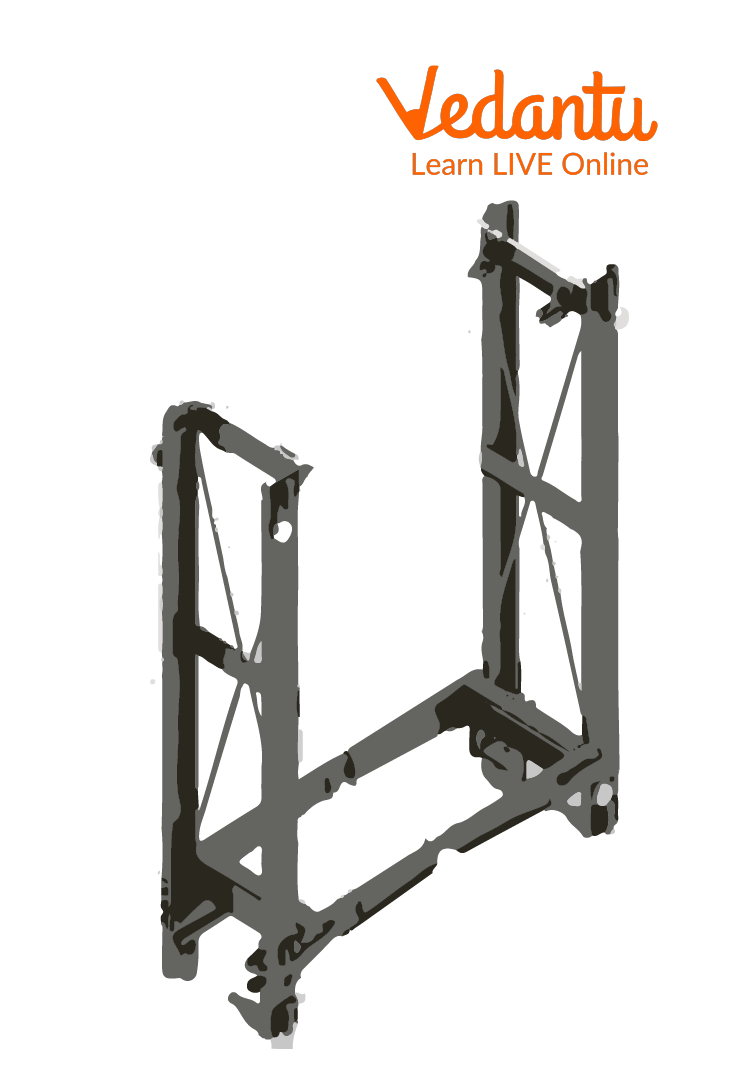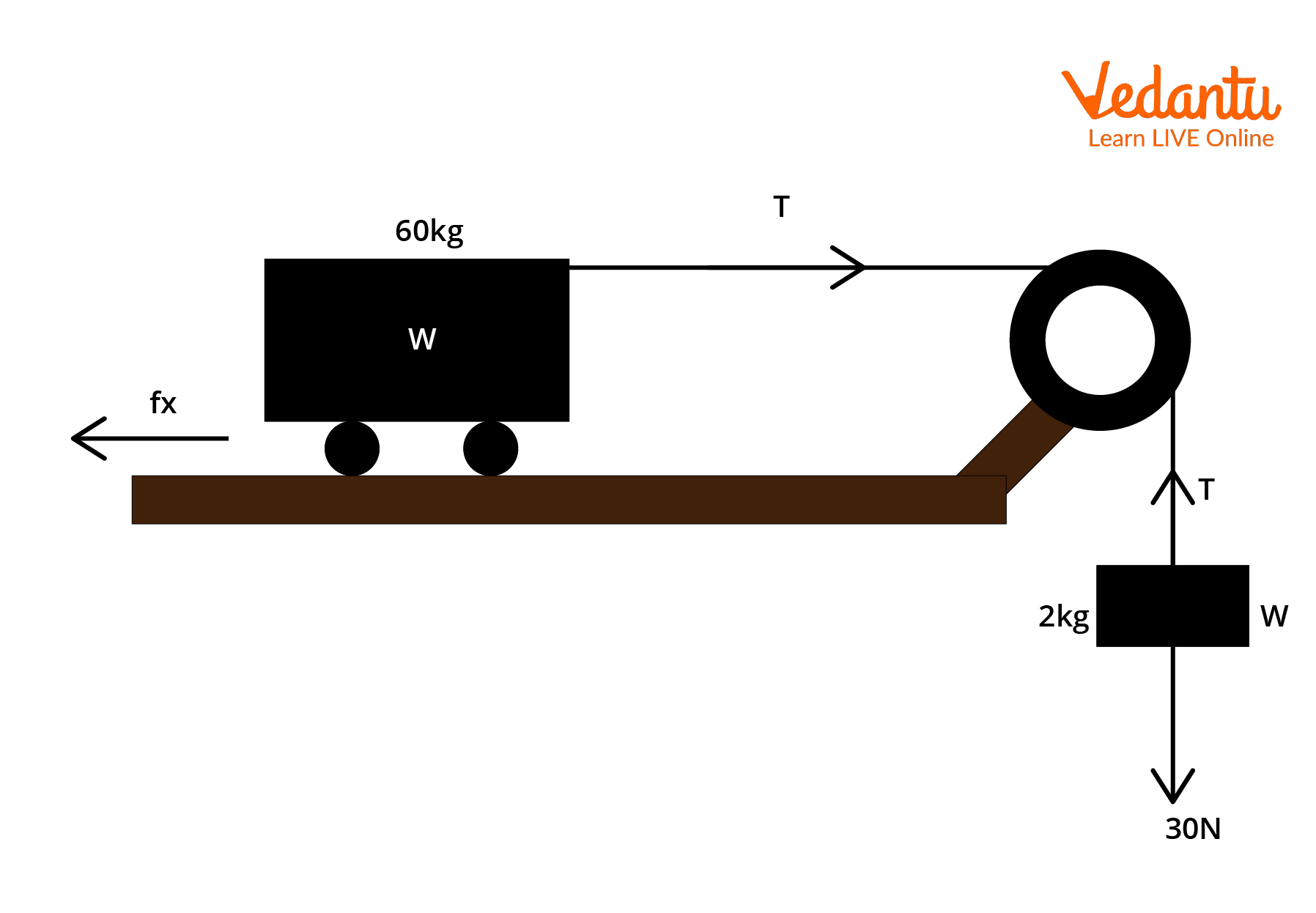Courses
Courses for Kids
Free study material
Free LIVE classes
More

# Movable Pulley and Its Solved Examples for JEELIVE
Join Vedantu’s FREE Mastercalss

## An Introduction to Movable Pulley

What do elevators, weight lifting machines, construction equipment etc., have in common? It is a pulley. Pulleys are nothing but a wheel fixed on an axle or shaft that causes movement. A pulley is free to move up or down and may be attached to the ceiling or any other object. Aside from its constructional details a pulley system has a major advantage that it eases the work of human beings from carrying heavy loads.

Additionally, they are also easier to manufacture, are cheap and require no lubrication.As per the type of application pulleys are further divided into movable pulleys, fixed pulleys, compound, block-tackle and compound pulleys etc. Thus, there are several pulleys out there and each one of them have their specific uses. Let us look at some examples below to understand the different types of pulleys!

## What is a Movable Pulley?

Now that we know what a  pulley system is, let us understand one of its types, i.e a movable pulley. Refer to the figure below. For a movable pulley one end of the rope is fixed and the other one left free to attach a load. They are attached to the ceiling or any other object. This type of pulley helps to lift loads from a lower to higher level. For example, a painter trying to fetch a paint box from the lower end of the building or construction workers trying to carry stones to a higher level.Image of a movable pulley

A movable pulley can also act as a wheel and axle. If both ends of the rope are fixed then the object to be lifted or moved willmove along the rope.

## Relationship between Tension and the Acceleration Acting on a BodyImage of a pulley system that consists of a fixed and movable pulley

Consider two masses m1 and m2 connected to a pulley system as shown in the figure below. If the weight acting on a movable pulley is moving with a downward acceleration a2 and the weight attached to the fixed pulley is moving with upward acceleration a1, then how do we determine the relationship between upward and downward acceleration.

The tension force acting is as shown in the figure below. Using the constraint relations equations we have $\sum T . a=0$ or $\sum T a \cos (\theta)=0$

The constraint relations equation states that the summation of all tension forces acting on the string and the accelerations acting on the body is equal to zero. The strings in such a case must be inextensible i.e. of the same length and tight.

As the angle between T and a1 is zero therefore we write the above equation as:

\begin{align} &T a_{1} \cos (\theta) \\ &=T a_{1} \cos (0) \\ &=T a_{1} \end{align}

As the angle between T and a2 is 180 we write the above equation as

\begin{align} &2 T a_{2} \cos (\theta) \\ &=2 T a_{2} \cos \left(180^{\circ}\right) \\ &=-2 T a_{2} \end{align}

Adding the two we get the relationship between the two accelerations as:

\begin{align} &T a_{1}-2 T a_{2}=0 \\ &\Rightarrow T a_{1}=2 T a_{2} \\ &\Rightarrow a_{1}=2 a_{2} \end{align}

Additionally, it must also be known that with the help of a pulley the force required to lift a mass m is half as to the force required to lift it without a pulley system. It is denoted as $F=\dfrac{m g}{2}$. By using a pulley the force required is separated into tension forces denoted by T. Refer to the figure below.Distribution of tension forces

## Examples of a Movable Pulley

Some of the examples of a movable pulley include: construction equipment, zip lining, climbing pulley, cargo lift and so on.

### 1. Rock Climbing Pulleys

As the name suggests, Climbing pulley is usually employed when a person wishes to climb a mountain or tree. Sports like rock climbing employ climbing pulleys where the person who climbs acts as a load. As the person pulls the other end of the rope he/she starts ascending.

2. Cargo Lift or Hydraulic LiftsCargo or Hydraulic Lift

Cargo lifts also known as hydraulic lifts are specifically used in industries to carry people or load from one floor to another.

### 3. Construction Site Equipment

Movable pulleys are a common use in construction sites where the raw materials are to be transported from one floor to another. It requires almost half the effort to lift heavy loads from floor to another,thus movable pulleys are a common use at the construction sites.

### 4. Zip-line or Zip-wireZip-line or Zip-wire

Zip lining is an adventurous sport done in hilly areas. The person zip lining has to incline down from the top of the cable to its bottom. The person glides with the help of a freely movable pulley. Gravity also plays its part and aids the person to incline down. Thus, only half the effort is required by the movable pulley.

## Single Movable Pulley

A single movable pulley consists of two pulleys one of which is fixed and the other one is movable. The movable one is free to move up and down and carries the load. The mechanical advantage of a movable pulley is 2 (in an ideal case), which in real sense means that the pulley requires only half the effort to move it. As one of the pulleys is fixed it absorbs half the force to move the load. Thus, this reduces the effort required by the movable pulley to lift the load. Some single movable pulley examples include: construction cranes, modern elevators, some weight lifting machines in the gym, the sails on the sailboats, and so on.

## Solved Examples for a Pulley System

Example 1: The figure below shows two pulleys one fixed and the other movable. Determine the velocity ratio and the mechanical advantage if a 300 N force is required to lift a  weight of 800 N.

Solution:Figure shows a fixed and a movable pulley

The velocity ratio is given as 2n. N here denoted the no. of movable pulleys connected to the fixed pulley. As the figure shows only one movable pulley connected therefore the velocity ratio is 2.

Mechanical advantage on the other hand is given as the ratio between load to effort. The load here is 800 N whereas the effort required is 300 N. Thus, ratio becomes  $\dfrac{800}{300}=\dfrac{8}{3}$

Example 2: What is the acceleration between a mass and a block W as shown in the figure below. Assume the coefficient of friction between the surface and the block W is 0.02. Also calculate the tension in the string? Assume g= 10 ms-1 and neglect the mass of the string.Figure of a block and a mass connected to a pulley

Solution:

As the weight of the block W and mass m is 60 kgs and 2 kgs respectively, are attached to a smoothless pulley and the string is inextensible the acceleration experienced by both the block W and the mass will be the same.

Applying the second law of motion to the block W and the mass m we get the equations as:

\begin{align} &20-T=2 a \ldots(1) \\ &T-f_{k}=60 a \ldots(2) \end{align}

Soving 1 and 2 we get,

$20-f_{k}=80 a$

Sliding friction is denoted as and the coefficient of sliding friction as $f_{k}=\mu_{k} N$

It is the force acting in opposition to the relative motion of surfaces in contact. It is independent of the contact area and has a relationship somewhat similar to static friction.

Substituting the value of coefficient of sliding friction above we get, $600 \times 0.02=12 \mathrm{~N}$

Putting the value of fk in (2) we get,

$T-12=60 a \ldots(3)$

Now, solving (1) and (3) we get,

\begin{align} &20-T=2 a \ldots(3) \\ &T-12=60 a \ldots(1) \\ &\Rightarrow 20-12=80 a \\ &\Rightarrow a=\dfrac{8}{80} \\ &\Rightarrow a=0.1 m s^{-2} \end{align}

Putting value of a in (3), we get

\begin{align} &20-T=2(0.1) \\ &20-T=0.2 \\ &T=19.8 \mathrm{~N} \end{align}

## Conclusion

As we have discussed the movable pulleys thoroughly, thus we can conclude that pulleys have certain advantages. Their advantages include they provide mechanical advantage, noiseless lifting, change the direction of applied force and are cheap. Even though movable pulleys work well for some applications thus, besides the numerous advantages there are also certain disadvantages like the rope may creep due to the prolonged forces being applied on it,  there is a possibility of sli and they cannot be employed for a very high power transfer. A pulley system has its uses but also has some cons that need to be addressed.

Last updated date: 18th Sep 2023
Total views: 132.9k
Views today: 3.32k

## FAQs on Movable Pulley and Its Solved Examples for JEE

1. What is pulley? State some pulley examples.

A pulley essentially consists of a  wheel which is supported by an axle or a shaft. The axle or the shaft is used to support the movement, change direction or transfer power between the shaft and the cable or belt it is connected to.Some pulley example include: a well to lift the bucket of water out the well an elevator, a cargo lift, cranes,exercise equipments, and so on. Pulleys are further classified into a single pulley system, movable pulleys and compound pulleys.

2. What does mechanical advantage of a pulley mean?

The ratio of load to effort is the mechanical advantage of a pulley. It is denoted as $\text { Mechanical Advantage }=\dfrac{\text { Load }}{\text { Effort }}$. The higher the mechanical advantage the lesser the effort will be required by the pulley to lift heavy objects. For example the single movable pulley has a mechanical advantage of 2 for an ideal case. Practically, it may vary between 1 to 2. For a single pulley that is fixed to the ceiling the mechanical advantage is 1. Thus, movable pulleys are more advantageous than fixed pulleys. Fixed pulleys or single pulleys only help to change the direction of force applied to lift the load.